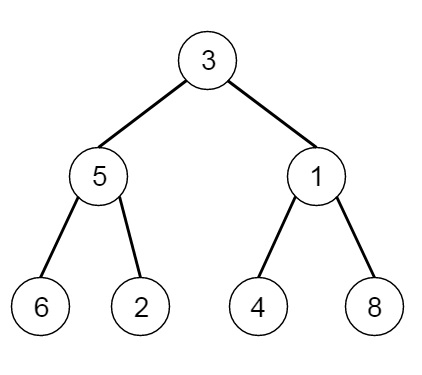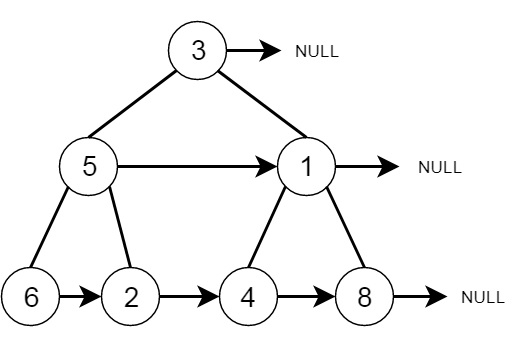# Populating Next Right Pointers in Each Node in C++

C++Server Side ProgrammingProgramming

Suppose we have a complete binary tree, where each node has following fields: (data, left, right, next), the left will point to left subtree, right will point to right subtree, and the next pointer will point to the next node. If there is no node in the right hand side, then that will be null. So initially each next pointer is set to null, we have to make the links. Suppose the tree is like the first one, it will be converted to the next node −To solve this, we will follow these steps −

• set pre := root, nextPre := null and prev := null
• while pre is not null
• while pre is not null
• if left of pre is not null
• set next of prev := left of pre, when prev is not null, otherwise nextPre := left of pre
• prev := left of pre
• if right of pre is not null
• set next of prev := right of pre, when prev is not null, otherwise nextPre := right of pre
• prev := right of pre
• pre := nextPre
• set nextPre as null and prev as null
• return null

Let us see the following implementation to get better understanding −

## Example

Live Demo

#include <bits/stdc++.h>
#include <stack>
using namespace std;
class Node {
public:
int val;
Node* left;
Node* right;
Node* next;
Node() {}
Node(int _val, Node* _left, Node* _right) {
val = _val;
left = _left;
right = _right;
next = NULL;
}
};
class Solution {
public:
Node* connect(Node* root) {
Node* pre = root;
Node* nextPre = NULL;
Node* prev = NULL;
while(pre){
while(pre){
//cout << pre->val << endl;
if(pre->left){
if(prev){
prev->next = pre->left;
}else{
nextPre = pre->left;
}
prev = pre->left;
}
if(pre->right){
if(prev){
prev->next = pre->right;
}else{
nextPre = pre->right;
}
prev = pre->right;
}
pre = pre->next;
}
//cout << "*" << endl;
pre = nextPre;
nextPre = NULL;
prev = NULL;
}
return root;
}
};
void printTree(Node* root) {
cout << "[";
if (root == NULL) return;
queue<Node*> q;
Node *curr;
q.push(root);
q.push(NULL);
while (q.size() > 1) {
curr = q.front();
q.pop();
if (curr == NULL){
q.push(NULL);
}
else {
// if(curr->next)
// q.push(curr->next);
if(curr->left)
q.push(curr->left);
if(curr->right)
q.push(curr->right);
if(curr->val == 0){
cout << "null" << ", ";
}else{
cout << curr->val << ", ";
if (curr->next == NULL) cout<<"#, ";
}
}
}
cout << "]"<<endl;
}
int main() {
Node* root;
Node nodeFour(4, NULL, NULL);
Node nodeFive(5, NULL, NULL );
Node nodeSeven(7, NULL, NULL);
Node nodeSix(6, NULL, NULL);
Node nodeTwo(2,&nodeFour,&nodeFive);
Node nodeThree(3,&nodeSix,&nodeSeven);
Node nodeOne(1,&nodeTwo,&nodeThree);
root = &nodeOne;
Solution ob;
root = ob.connect(root);
printTree(root);
}

## Input

[1,2,3,4,5,6,7]
Node* root;
Node nodeFour(4, NULL, NULL);
Node nodeFive(5, NULL, NULL );
Node nodeSeven(7, NULL, NULL);
Node nodeSix(6, NULL, NULL);
Node nodeTwo(2,&nodeFour,&nodeFive);
Node nodeThree(3,&nodeSix,&nodeSeven);
Node nodeOne(1,&nodeTwo,&nodeThree);
root = &nodeOne;
Solution ob;
root = ob.connect(root);

## Output

[1, #, 2, 3, #, 4, 5, 6, 7, #, ]